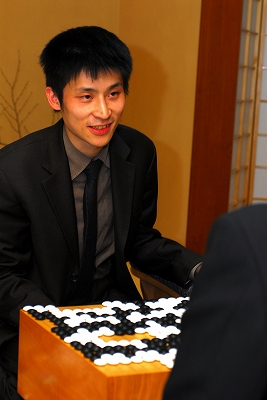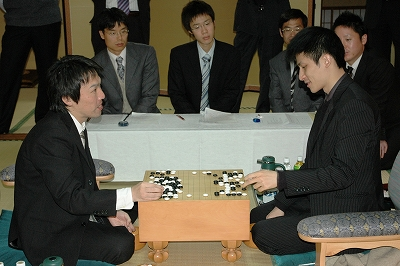Posts Tagged ‘oza’

Cho U Keeps Oza Title

Thursday, November 26th, 2009

Cho U defeated challenger Yamada Kimio with 3 straight wins and kept his Oza title for another year.The 3rd game record is here. Cho U (white) won by 3.5 points.

(; KM[6.5] EV[57th Oza title match 3] FF SZ GM PW[Cho U] WR[Oza] DT[2009-11-26] BR[9d] RE[W+3.5] PB[Yamada Kimio] ;B[pd];W[dd];B[pq];W[dq];B[do];W[cm];B[cq];W[gq];B[dp];W[dr];B[bo] ;W[cj];B[ip];W[cr];B[gp];W[fp];B[fo];W[ep];B[eo];W[hp];B[go];W[hq] ;B[ho];W[iq];B[jp];W[jq];B[kp];W[lq];B[ch];W[ej];B[eh];W[gc];B[gi] ;W[cf];B[ic];W[gk];B[ie];W[qf];B[qh];W[kc];B[ge];W[nc];B[pc];W[ph] ;B[pi];W[pg];B[qj];W[ne];B[re];W[qe];B[rd];W[nh];B[dl];W[el];B[bd] ;W[cc];B[ce];W[de];B[bf];W[dm];B[po];W[qm];B[qo];W[ri];B[qi];W[nj] ;B[pl];W[nl];B[pm];W[fh];B[fi];W[ei];B[eg];W[ff];B[fg];W[gf];B[ke] ;W[cd];B[be];W[ef];B[bi];W[ig];B[md];W[nd];B[lb];W[ob];B[rf];W[jb] ;B[lc];W[ib];B[kd];W[lf];B[jg];W[le];B[ld];W[jf];B[hg];W[jh];B[hf] ;W[gg];B[kg];W[if];B[hh];W[kf];B[gh];W[hc];B[id];W[bq];B[hl];W[gl] ;B[hm];W[jj];B[mr];W[lr];B[kl];W[cg];B[bg];W[bj];B[bc];W[bb];B[ab] ;W[cb];B[fd];W[fc];B[lk];W[hk];B[ki];W[ji];B[ai];W[il];B[im];W[jl] ;B[jm];W[or];B[mi];W[ni];B[mq];W[lp];B[np];W[ko];B[ln];W[lo];B[mn] ;W[no];B[mo];W[mp];B[nq];W[kn];B[km];W[nn];B[ml];W[ol];B[kh];W[pk] ;B[qk];W[on];B[mm];W[pn];B[ok];W[nk];B[qn];W[jn];B[mg];W[mh];B[lg] ;W[ng];B[mf];W[me];B[in];W[oj];B[ql];W[ms];B[ns];W[ls];B[ih];W[pb] ;B[qb];W[rb];B[qc];W[qa];B[rc];W[qg];B[rg];W[kj];B[lj];W[hj];B[nr] ;W[op];B[oq];W[oo];B[pp];W[cp];B[co];W[fm];B[gm];W[fn];B[bm];W[bl] ;B[am];W[bp];B[ao];W[pe];B[ed];W[ec];B[kq];W[kr];B[io];W[kb];B[ka] ;W[je];B[jd];W[ac];B[ad];W[ci];B[dh];W[df];B[na];W[nb];B[bh];W[kk] ;B[oi];W[nf];B[li];W[pj];B[di];W[dj];B[fj];W[fk];B[al];W[ak];B[aj] ;W[bk];B[cn];W[ja];B[la];W[ra];B[sb];W[oh];B[en];W[em];B[ap];W[aq] ;B[od];W[oe];B[mk];W[ba];B[gn])

Complete tournament table is here.

Cho U – Yamada Kimio 2:0 in Oza Title

Wednesday, November 25th, 2009

Cho U, Oza title holder, won the second game as well against challenger Yamada Kimio. Cho U is just one win away from keeping this title for another year.The game record is here. Cho U (black) won by resignation.

(; DT[2009-11-24] PB[Cho U] RE[B+R] PW[Yamada Kimio] FF SZ GM BR[Oza] KM[6.5] EV[57th Oza title match 2] WR[9d] ;B[pd];W[dd];B[qp];W[dp];B[fq];W[hq];B[nq];W[qj];B[cn];W[fp];B[cq] ;W[dq];B[cp];W[do];B[cr];W[dr];B[fc];W[cf];B[db];W[qf];B[nd];W[ic] ;B[cc];W[lc];B[ld];W[md];B[me];W[mc];B[ne];W[kd];B[qe];W[qm];B[nc] ;W[rf];B[le];W[cl];B[dn];W[ci];B[jd];W[jc];B[ke];W[id];B[kc];W[kb] ;B[pm];W[ql];B[iq];W[ip];B[jp];W[hp];B[jr];W[jo];B[hr];W[kp];B[jq] ;W[mq];B[ko];W[np];B[oq];W[lp];B[gq];W[gp];B[eo];W[ep];B[jn];W[io] ;B[kn];W[lr];B[fs];W[cs];B[mo];W[mp];B[on];W[co];B[nr];W[po];B[oo] ;W[bo];B[qn];W[rn];B[qo];W[el];B[hm];W[rd];B[fm];W[em];B[be];W[ce] ;B[di];W[dh];B[ei];W[cj];B[oj];W[ph];B[pk];W[qk];B[pg];W[gk];B[ik] ;W[qh];B[bf];W[bg];B[oh];W[ii];B[hj];W[hh];B[cd];W[jj];B[jk];W[lj] ;B[kk];W[pl];B[ol];W[nk];B[ok];W[ni];B[oi];W[af];B[ie];W[gd];B[de] ;W[df];B[ee];W[gc];B[ef];W[eh];B[fh];W[gg];B[eg];W[ch];B[hi];W[ih] ;B[kj];W[ki];B[ge];W[fe];B[ff];W[he];B[fd];W[hf];B[rm];W[ro];B[rp] ;W[rl];B[qc];W[lh];B[dg];W[cg];B[lk];W[mk];B[mj];W[nj];B[li];W[mi] ;B[lj];W[mg];B[ml];W[rc];B[qb];W[nb];B[re];W[se];B[ob];W[fb];B[so] ;W[sm];B[bd];W[fg];B[fj];W[gf];B[dc];W[fe];B[ed];W[eb];B[ec];W[fn] ;B[dk];W[dj];B[ek])

The next game will be played on November 26th. Complete tournament table is here.

Cho U Wins First Game in Oza Title Match

Friday, October 30th, 2009

Cho U, title holder of the Oza title, defeated challenger Yamada Kimio in the first game of this best-of-five title match.

This title must be quite important for Cho U: while he is still dominating the Japanese Go by the number of title he is holding, his very recent loss of Meijin title to Iyama Yuta is a sign that Cho U may not be in top shape anymore.The game record is here.

(; EV[57th Oza title match 1] KM[6.5] FF SZ GM PW[Cho U] WR[Oza] DT[2009-10-23] BR[9d] RE[W+R] PB[Yamada Kimio] ;B[qd];W[dc];B[dp];W[pp];B[ce];W[ed];B[ci];W[oc];B[kd];W[pe];B[qe] ;W[pf];B[qg];W[qc];B[pg];W[pd];B[ng];W[ic];B[rc];W[qb];B[qn];W[nq] ;B[rp];W[kq];B[qq];W[pm];B[pn];W[om];B[qm];W[on];B[fq];W[bp];B[cp] ;W[bo];B[bq];W[br];B[cq];W[cl];B[bm];W[cm];B[bn];W[co];B[cn];W[do] ;B[dn];W[eo];B[gp];W[go];B[ho];W[em];B[dm];W[dl];B[bl];W[fp];B[hq] ;W[aq];B[cr];W[hn];B[io];W[ej];B[en];W[eq];B[ep];W[fo];B[er];W[fm] ;B[el];W[fl];B[ek];W[dj];B[fk];W[gk];B[fj];W[cj];B[bj];W[bi];B[ck] ;W[dk];B[bk];W[ch];B[fn];W[gn];B[gm];W[gl];B[hm];W[il];B[im];W[in] ;B[jm];W[jn];B[di];W[ei];B[dh];W[dg];B[eh];W[fi];B[gj];W[fh];B[ik] ;W[hj];B[gi];W[jl];B[km];W[gh];B[hi];W[ij])

The next game will be played on November 24th. Complete tournament table is here.

Cho U Captures Oza Title

Monday, December 8th, 2008

Just a few days after capturing the Tengen title, Cho U also captured the Oza title after defeating Yamashita Keigo 3-1.

Cho won the first two games in the title challenge, then Yamashita won game three. Game four was won again by Cho, by 2.5 points.Cho U owns now Meijin, Tengen, Gosei and Oza titles, which shows that he is the top player in Japan at the moment.

Here is the record of the 4th game in the Oza title match.

Yamashita Hangs on in Oza Title Defense

Monday, December 1st, 2008
Yamashita Keigo won game 3 of the Oza title match by 5.5 points, thus surviving in his title defense (since Oza title is played as a best-of-five match). Cho U won the first 2 games.Here is the game record.

Yamashita Defends Oza

Thursday, November 29th, 2007
Yamashita successfully defended the Oza title this year, against Imamura Toshiya: he won the 4th game today, so the final score is 3-1.

Oza Title: 2-1 for Yamashita Kisei

Tuesday, November 27th, 2007
Yamashita Keigo, who also ows the most prestigious Japanese Go Title, Kisei, took the lead again in his defense of the Oza title: he leads Imamura Toshiya by 2-1 after today’s win, and he is only one game away from winning this title match. White (Yamashita) managed a very clean and sharp cut at move 80, in a textbook style. Imamura must have been very sad to see his position split into two groups in such a painful way. It was all due to a very skillful series of three forcing / peeping moves by Yamashita. Check out the game record for details.Next game is in two days, on November 29th.